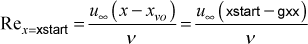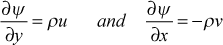# Institut für Thermodynamik der Luft- und Raumfahrt - Universität StuttgartMechanical Engineering - The University of Texas at Austin# integration control

The primary controls for integration of the transport equations include being able to define the physical flow domain for integration and the integration stepsize. For external flows, there are additional controls related to the growth of the boundary layer, because the external (E) surface is not a fixed location.

Please read the section overview: numerical accuracy to further understand how the user can affect the solution with respect to stepsize and grid.

Physical Flow Domain - The external flow two-dimensional (2D) flow domain lays against the surface and extends into the free stream. The axial extent of the flow domain is defined for TEXSTAN using the boundary condition array x(m) and there will be nxbc values within the array, where m = 1, nxbc. For example, flow over a flat plate will have a domain that typically extends from the plate leading edge to its trailing edge, and nxbc=2 could be used as a minimum if u is constant over the surface and the surface is either isothermal (constant temperature) or constant heat flux. If there is a need to specify a surface temperature distribution (or surface heat flux distribution) or u = u(x), then more boundary condition locations will be required.

The corresponding cross-flow extent of the domain extends from the surface (the I-surface) to the free stream (E-surface), often called the boundary layer edge. The extent of this domain is initially set by the last value of the initial velocity profile, u(y), where the profile is most often TEXSTAN-generated using kstart. For example, with the Blasius flow, the TEXSTAN-generated velocity profile extends such that about 10-20% of the profile is in the region where u=u. As integration proceeds, TEXSTAN carefully monitors the u(y) profile near the E-surface and when it begins to develop a significant non-zero gradient, TEXSTAN expands the extent of the cross-stream domain to ensure a small-to-zero velocity gradient, using a process called entrainment. Note that TEXSTAN also ensures near-zero gradients of other transport variables, depending on the choice of the kent variable.

Integration Start and Stop Locations - These are defined by the xstart and xend variables. The starting location of the integration is xstart and the ending location of the integration is xend. The only restrictions are that they be bound within the boundary condition array x(m). It is permissible to have x(1) be negative. For example, in modeling an isentropic supersonic nozzle, the throat location could arbitrarily be set x=0, such that locations in the upstream nozzle section are negative x values. The starting x-Reynolds number is formed from xstartwhere xvo is the virtual origin, set =0 by default in TEXSTAN, or input using k1 and gxx. The starting x-Reynolds number is only used for convenience in various output files.

There is one restriction on xstart for external flows that is different from internal flows. For internal flows, the numerical grid is required to extend from the surface to the centerline for pipes and thermally-symmetrical parallel-planes channels (kgeom=4,5) or from surface to surface for thermally-asymmetrical parallel-planes channels and annuli (kgeom=6,7). For external flows, the numerical grid extends only from the surface to just above the shear layer (boundary layer). This is usually called the free stream. Because of the nature of the transformed equations in TEXSTAN, the computations can not start with a zero-thickness boundary layer or from a finite thickness boundary layer that has a free stream velocity of zero (the 2-D and axisymmetric stagnation point problem). These constraints are never violated for internal flow problems.

Therefore, for external flows only, it is required that xstart not be at the leading edge of a flat plate or at a surface stagnation point. A suggested value is to back-calculate a value for xstart by choosing a starting x-Reynolds number (1000 is sufficiently small to ensure laminar flow for any transitional flows such as flow over a turbine blade).

Integration Stepsize - This is primarily controlled by deltax, and alternatively by the kdx flag along with the aux1(m) variable array. Integration stepsize in TEXSTAN is proportional to the boundary layer thickness, so as the boundary layer grows, the stepsize automatically increases. The primary input variable for stepsize control is deltax. This value is monitored to insure that the integration stepsize does not grow too large for the case of near-separated flows. We typically set deltax =0.05 to =0.10, and this works well for most laminar, transitional, and turbulent flows.

It is the responsibility of the user to keep the integration stepsize small to resolve fast-changing boundary conditions, because TEXSTAN does not have an auto-stepsize feature. For example, if the wall heat flux over the first 50% of a flat plate problem is adiabatic, and the last 50% is a prescribed heat flux for heating or cooling, then numerical accuracy for resolving the fast rate of change of the surface temperature immediately after the step-change in the surface heat flux would require a very small stepsize close to the step and the stepsize could be increased further downstream. TEXSTAN has a feature to manually control this stepsize (again, it is not automatic). To provide more control over stepsize, the flag kdx can be set =1. When this flag is switched on, the stepsize variable deltax is set =0, and in its place, integration stepsize information is input using the array aux1(m). This array is then linearly interpolated as a function of x, and the resulting value has precisely the same meaning as deltax.

Entrainment Control - For external flows, the region above the wall that is influenced by the wall boundary conditions varies in the flow direction, and its extent is different for each transport equation (velocity, temperature or stagnation enthalpy, turbulence kinetic energy, and so forth). That is, each equation has its own boundary layer thickness, defined as the location where the dependent variable is within, say, 1 percent of its free stream value. The finite difference computational domain must extend beyond the largest of the boundary layer thicknesses to maintain an overall grid- or finite-difference mesh-independent solution.

Entrainment in TEXSTAN effectively enlarges the region between the I- and E-surface because of the nature of the TEXSTAN cross-stream variable. Note that for other types of finite-difference programs, the computations have to be stopped, a new mesh constructed, and the variable values interpolated onto the new grid, before integration can continue. Note that for internal flows have a computational domain that is fixed and therefore, entrainment does not have to be considered.

A set of three variables in TEXSTAN are used to control entrainment for external flows: enfra, fra, and kent. In summary, the overall entrainment procedure at each integration step is to compute the near-edge gradient of each dependent variables within the set of variables defined by the value of kent. For example, kent=0 requires only the edge velocity gradient to be tested, and kent=1 requires additional testing of the temperature (or stagnation enthalpy) profile. Each tested gradient is then made nondimensional and compared with the value of enfra. If the absolute value of any tested gradient exceeds the enfra value, an algorithm computes an incremental E-surface mass flux and adds it to the existing E-surface value. The incremental mass flux is then tested against the fra value and limited to the fra value.

Entrainment Theory - External flows are flows in which one boundary condition is a wall and the other boundary condition is the ill-defined "infinity" boundary condition. Technically we call this type of flow an external wall shear flow, and we call flows with two "infinity" boundary conditions external free shear flows (jets, wakes, and mixing layers). For either case there are two mathematical boundary conditions that must be satisfied at "infinity", namely the free stream value and a zero gradient. Thus, boundary layer flows (external flows) have to have special handling for the "infinite" boundary condition to insure that all dependent variable gradients (velocity, temperature, turbulence kinetic energy, and so forth) approach zero as the dependent variable values approach their free stream values.

Internal flows, on the other hand, are flows in which the dependent variable (velocity, temperature, turbulence kinetic energy, and so forth) have boundary conditions at the boundaries of the computational flow domain that are completely satisfied mathematically. For example, in a pipe flow, the dependent variable value (Dirichlet) or flux (Neumann) is specified at a wall and a zero gradient (Neumann) at a symmetry line. This is primarily why computational fluid dynamics modeling (CFD) are attracted to these domains.

For zero pressure gradient and adverse pressure gradient boundary layers, the boundary layer grows (the boundary layer thickness, δ99, increases) as the flow moves in the x-direction. If the edge of the boundary layer is not allowed to physically expand outward into the free stream, the edge velocity will still be (forced) equal to the free stream value, but the gradient will move away from zero and become positive, violating the zero-gradient condition. The converse will happen if the flow accelerates, because the boundary layer becomes thinner.

The external boundary layer "infinity" boundary condition problem is independent of whether the cross-stream coordinate is dimensional (y) or dimensionless. The Blasius variable is often used, or the stream function variable, associated with the von Mises transformation, or in the case of TEXSTAN, a nondimensional form of the stream function, called the Patankar-Spalding ω variable - see the overview: transport equations section of this website. The computational grid has to either be so large in the cross-stream direction that for all x-locations, the "infinity" problem is not violated, or the grid is small (to avoid wasted computations) and expanded as the flow proceeds in the x direction.

The concept of entrainment is most easily seen if we transform the velocity components using the two-dimensional compressible stream function,Only at "infinity" will (∂ψ/∂x) → 0 and the value of "infinity" is not really known if y or ψ is selected as the cross-stream variable. If the Blasius variable is chosen, then the problem is more easily solved because η of about 7-8 is "infinity" if there is no pressure gradient.

website updated Sept 2010   © 1996-2010 Michael E. Crawford - all rights reserved - website validated for CSS 2.1 and XHTML 1.0 strict at www.w3.org